Thursday 21st January 2021

CBSE Guess > Papers > Question Papers > Class XII > 2004 > Maths > Delhi Set-III

MATHS (Set III—Delhi)

Except for the following questions, all the remaining questions have been asked in Set I and Set II.

SECTION - A

Q. 2. An urn contains 6 white, 4 black and 5 red balls. Two balls are drawn at random. Find the probability that (i) both the balls are red
(ii) one ball is red, the other is black
(iii) one ball is white

Q. 4. Using properties of determinants, Prove that: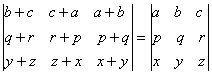Q. 7. Evaluate: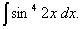Q. 13. Prove that the curves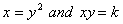and cut at right angles if 8K2 =1.

Or

Verify Lagrange's mean value theorem for the function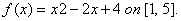Q. 14. Evaluate: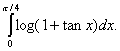Q. 18. Using matrix method solve the following system of linear equations:

SECTION - B

Q. 21 . Find x such that the four points A (3, 2, 1) B (4, x, 5) C (4, 2, -2) and D (6, 5, -1) coplanar.

Or

Prove that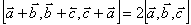SECTION - C

Q. 22. Three urns A, B and C contain 4 red and 6 white; 3 red and 5 white; and 2 red and 4 white balls respectively. An urn is chosen at random and a ball is drawn. If the ball drawn is fond to be red, find the probability that the ball was drawn from urn A.

 Maths 2004 Question Papers Class XII Delhi Outside Delhi Compartment Delhi Compartment Outside DelhiSet ISet ISet ISet ISet IISet IISet IISet IISet IIISet III

CBSE 2004 Question Papers Class XII